• function [I_SSD,I_NCC]=template_matching(T,I)% TEMPLATE_MATCHING is a cpu efficient function which calculates matching% score images between template and an (color) 2D or 3D image.% It calculates:% - ...

function [I_SSD,I_NCC]=template_matching(T,I)
% TEMPLATE_MATCHING is a cpu efficient function which calculates matching
% score images between template and an (color) 2D or 3D image.
% It calculates:
% - The sum of squared difference (SSD Block Matching), robust template
%   matching.
% - The normalized cross correlation (NCC), independent of illumination,
%   only dependent on texture
% The user can combine the two images, to get template matching which
% works robust with his application.
% Both measures are implemented using FFT based correlation.
%
% [I_SSD,I_NCC]=template_matching(T,I)
% inputs,
%   T : Image Template, can be grayscale or color 2D or 3D.
%   I : Color image, can be grayscale or color 2D or 3D.
%
% outputs,
%   I_SSD: The sum of squared difference 2D/3D image. The SSD sign is
%          reversed and normalized to range [0 1]
%   I_NCC: The normalized cross correlation 2D/3D image. The values
%          range between 0 and 1
%
% Example 2D,
%   % Find maximum response
%   % Template of Eye Lena
%    T=I(124:140,124:140,:);
%   % Calculate SSD and NCC between Template and Image
%    [I_SSD,I_NCC]=template_matching(T,I);
%   % Find maximum correspondence in I_SDD image
%    [x,y]=find(I_SSD==max(I_SSD(:)));
%   % Show result
%    figure,
%    subplot(2,2,1), imshow(I); hold on; plot(y,x,'r*'); title('Result')
%    subplot(2,2,2), imshow(T); title('The eye template');
%    subplot(2,2,3), imshow(I_SSD); title('SSD Matching');
%    subplot(2,2,4), imshow(I_NCC); title('Normalized-CC');
%
% Example 3D,
%   % Make some random data
%    I=rand(50,60,50);
%   % Get a small volume as template
%    T=I(20:30,20:30,20:30);
%   % Calculate SDD between template and image
%    I_SSD=template_matching(T,I);
%   % Find maximum correspondence
%    [x,y,z]=ind2sub(size(I_SSD),find(I_SSD==max(I_SSD(:))));
%    disp(x);
%    disp(y);
%    disp(z);
%
% Function is written by D.Kroon University of Twente (August 2009)
% Convert images to double
T=double(T); I=double(I);
if(size(T,3)==3)
% Color Image detected
[I_SSD,I_NCC]=template_matching_color(T,I);
else
% Grayscale image or 3D volume
[I_SSD,I_NCC]=template_matching_gray(T,I);
end
function [I_SSD,I_NCC]=template_matching_color(T,I)
% Splite color image, and do template matching on R,G and B image
[I_SSD_R,I_NCC_R]=template_matching_gray(T(:,:,1),I(:,:,1));
[I_SSD_G,I_NCC_G]=template_matching_gray(T(:,:,2),I(:,:,2));
[I_SSD_B,I_NCC_B]=template_matching_gray(T(:,:,3),I(:,:,3));
% Combine the results
I_SSD=(I_SSD_R+I_SSD_G+I_SSD_B)/3;
I_NCC=(I_NCC_R+I_NCC_G+I_NCC_B)/3;
function [I_SSD,I_NCC]=template_matching_gray(T,I)
% Calculate correlation output size  = input size + padding template
T_size = size(T); I_size = size(I);
outsize = I_size + T_size-1;
% calculate correlation in frequency domain
if(length(T_size)==2)
FT = fft2(rot90(T,2),outsize(1),outsize(2));
FI = fft2(I,outsize(1),outsize(2));
Icorr = real(ifft2(FI.* FT));
else
FT = fftn(rot90_3D(T),outsize);
FI = fftn(I,outsize);
Icorr = real(ifftn(FI.* FT));
end
% Calculate Local Quadratic sum of Image and Template
LocalQSumI= local_sum(I.*I,T_size);
QSumT = sum(T(:).^2);
% SSD between template and image
I_SSD=LocalQSumI+QSumT-2*Icorr;
% Normalize to range 0..1
I_SSD=I_SSD-min(I_SSD(:));
I_SSD=1-(I_SSD./max(I_SSD(:)));
if (nargout>1)
% Normalized cross correlation STD
LocalSumI= local_sum(I,T_size);
% Standard deviation
stdI=sqrt(max(LocalQSumI-(LocalSumI.^2)/numel(T),0) );
stdT=sqrt(numel(T)-1)*std(T(:));
% Mean compensation
meanIT=LocalSumI*sum(T(:))/numel(T);
I_NCC= 0.5+(Icorr-meanIT)./ (2*stdT*max(stdI,stdT/1e5));
end
function T=rot90_3D(T)
T=flipdim(flipdim(flipdim(T,1),2),3);
Bstart=ceil((size(A)-Bsize)/2)+1;
Bend=Bstart+Bsize-1;
if(ndims(A)==2)
B=A(Bstart(1):Bend(1),Bstart(2):Bend(2));
elseif(ndims(A)==3)
B=A(Bstart(1):Bend(1),Bstart(2):Bend(2),Bstart(3):Bend(3));
end
function local_sum_I= local_sum(I,T_size)
% Calculate for each pixel the sum of the region around it,% with the region the size of the template.if(length(T_size)==2)    % 2D localsum    s = cumsum(B,1);    c = s(1+T_size(1):end-1,:)-s(1:end-T_size(1)-1,:);    s = cumsum(c,2);    local_sum_I= s(:,1+T_size(2):end-1)-s(:,1:end-T_size(2)-1);else    % 3D Localsum    s = cumsum(B,1);    c = s(1+T_size(1):end-1,:,:)-s(1:end-T_size(1)-1,:,:);    s = cumsum(c,2);    c = s(:,1+T_size(2):end-1,:)-s(:,1:end-T_size(2)-1,:);    s = cumsum(c,3);    local_sum_I  = s(:,:,1+T_size(3):end-1)-s(:,:,1:end-T_size(3)-1);end

展开全文• function [I_SSD,I_NCC]=template_matching(T,I)% TEMPLATE_MATCHING is a cpu efficient function which calculates matching % score images between template and an (color) 2D or 3D image.% It calculates:%
 function [I_SSD,I_NCC]=template_matching(T,I)% TEMPLATE_MATCHING is a cpu efficient function which calculates matching % score images between template and an (color) 2D or 3D image.% It calculates:% - The sum of squared difference (SSD Block Matching), robust template%   matching.% - The normalized cross correlation (NCC), independent of illumination,%   only dependent on texture% The user can combine the two images, to get template matching which% works robust with his application. % Both measures are implemented using FFT based correlation.%% [I_SSD,I_NCC]=template_matching(T,I)% inputs,%   T : Image Template, can be grayscale or color 2D or 3D.%   I : Color image, can be grayscale or color 2D or 3D.%% outputs,%   I_SSD: The sum of squared difference 2D/3D image. The SSD sign is%          reversed and normalized to range [0 1] %   I_NCC: The normalized cross correlation 2D/3D image. The values%          range between 0 and 1%% Example 2D,%   % Find maximum response%    I = im2double(imread('lena.jpg'));%   % Template of Eye Lena%    T=I(124:140,124:140,:);%   % Calculate SSD and NCC between Template and Image%    [I_SSD,I_NCC]=template_matching(T,I);%   % Find maximum correspondence in I_SDD image%    [x,y]=find(I_SSD==max(I_SSD(:)));%   % Show result%    figure, %    subplot(2,2,1), imshow(I); hold on; plot(y,x,'r*'); title('Result')%    subplot(2,2,2), imshow(T); title('The eye template');%    subplot(2,2,3), imshow(I_SSD); title('SSD Matching');%    subplot(2,2,4), imshow(I_NCC); title('Normalized-CC');%% Example 3D,%   % Make some random data%    I=rand(50,60,50);%   % Get a small volume as template%    T=I(20:30,20:30,20:30);%   % Calculate SDD between template and image%    I_SSD=template_matching(T,I);%   % Find maximum correspondence%    [x,y,z]=ind2sub(size(I_SSD),find(I_SSD==max(I_SSD(:))));%    disp(x);%    disp(y);%    disp(z);%% Function is written by D.Kroon University of Twente (August 2009)
% Convert images to doubleT=double(T); I=double(I);if(size(T,3)==3)     % Color Image detected    [I_SSD,I_NCC]=template_matching_color(T,I);else    % Grayscale image or 3D volume    [I_SSD,I_NCC]=template_matching_gray(T,I);end
function [I_SSD,I_NCC]=template_matching_color(T,I)% Splite color image, and do template matching on R,G and B image[I_SSD_R,I_NCC_R]=template_matching_gray(T(:,:,1),I(:,:,1));[I_SSD_G,I_NCC_G]=template_matching_gray(T(:,:,2),I(:,:,2));[I_SSD_B,I_NCC_B]=template_matching_gray(T(:,:,3),I(:,:,3));% Combine the resultsI_SSD=(I_SSD_R+I_SSD_G+I_SSD_B)/3;I_NCC=(I_NCC_R+I_NCC_G+I_NCC_B)/3;
function [I_SSD,I_NCC]=template_matching_gray(T,I)% Calculate correlation output size  = input size + padding templateT_size = size(T); I_size = size(I);outsize = I_size + T_size-1;
% calculate correlation in frequency domainif(length(T_size)==2)    FT = fft2(rot90(T,2),outsize(1),outsize(2));    FI = fft2(I,outsize(1),outsize(2));    Icorr = real(ifft2(FI.* FT));else    FT = fftn(rot90_3D(T),outsize);    FI = fftn(I,outsize);    Icorr = real(ifftn(FI.* FT));end
% Calculate Local Quadratic sum of Image and TemplateLocalQSumI= local_sum(I.*I,T_size);QSumT = sum(T(:).^2);
% SSD between template and imageI_SSD=LocalQSumI+QSumT-2*Icorr;
% Normalize to range 0..1I_SSD=I_SSD-min(I_SSD(:)); I_SSD=1-(I_SSD./max(I_SSD(:)));
if (nargout>1)    % Normalized cross correlation STD    LocalSumI= local_sum(I,T_size);    % Standard deviation    stdI=sqrt(max(LocalQSumI-(LocalSumI.^2)/numel(T),0) );    stdT=sqrt(numel(T)-1)*std(T(:));    % Mean compensation    meanIT=LocalSumI*sum(T(:))/numel(T);    I_NCC= 0.5+(Icorr-meanIT)./ (2*stdT*max(stdI,stdT/1e5));
function T=rot90_3D(T)T=flipdim(flipdim(flipdim(T,1),2),3);
% Calculate for each pixel the sum of the region around it,% with the region the size of the template.if(length(T_size)==2)    % 2D localsum    s = cumsum(B,1);    c = s(1+T_size(1):end-1,:)-s(1:end-T_size(1)-1,:);    s = cumsum(c,2);    local_sum_I= s(:,1+T_size(2):end-1)-s(:,1:end-T_size(2)-1);else    % 3D Localsum    s = cumsum(B,1);    c = s(1+T_size(1):end-1,:,:)-s(1:end-T_size(1)-1,:,:);    s = cumsum(c,2);    c = s(:,1+T_size(2):end-1,:)-s(:,1:end-T_size(2)-1,:);    s = cumsum(c,3);    local_sum_I  = s(:,:,1+T_size(3):end-1)-s(:,:,1:end-T_size(3)-1);end

展开全文image function fft plot
• MATLAB7.0编写，包含完整字符库及两张识别效果良好的车牌原图。基于模板匹配的简单实现，写了个GUI。7.0以上版本需修改fileparts函数的参数方可运行。识别算法是改进了一位博主的源码。
• 模板匹配 模板匹配实战 模板匹配 让我们继续探索使用过滤器作为模板的想法，我们想在二维空间中找到什么。 模板是你给我的东西，你说我想让你找到类似这样的东西，我们要通过过滤来做。 我想这是Christian ...
目录
模板匹配
模板匹配实战

模板匹配
让我们继续探索使用过滤器作为模板的想法，我们想在二维空间中找到什么。
模板是你给我的东西，你说我想让你找到类似这样的东西，我们要通过过滤来做。
我想这是Christian Grumman的一个很好的例子。
这里有一个非常简单的例子。如图：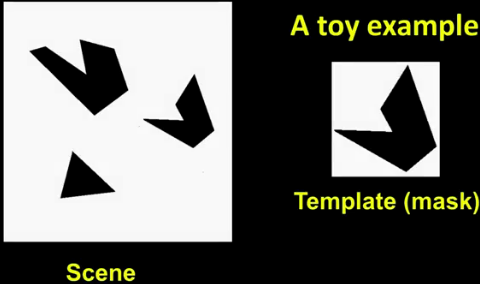左边是一个场景，右边是这个模板。
这就是我们想要找到的想法。
你看这里，你说，哦，我看到它在哪里了，它在，它在，它在这里。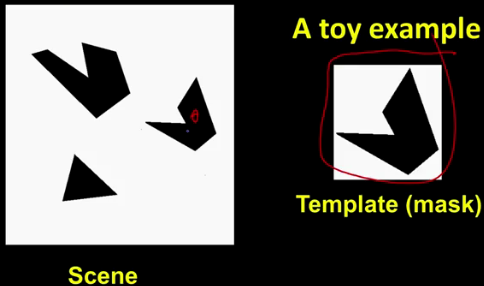我们想要的是系统吐出这样的东西。啊哈，这是我的绿色小盒子。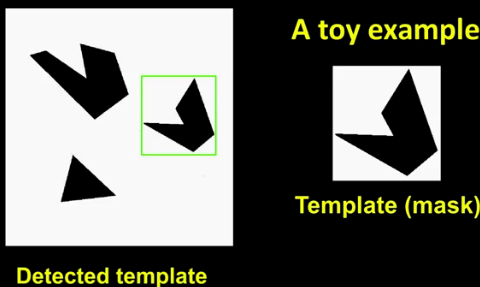我们能做的是我们可以通过归一化相关来做。
如果我使用这个带掩码的内核模板并与左边的图像进行归一化相关，我得到的是一个像这样的映射或相关图像：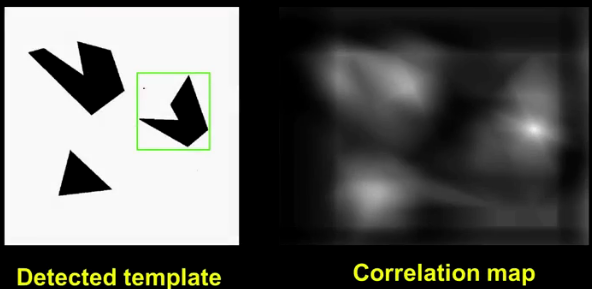你会注意到：黑色比上黑色，白色比上白色，你可以把它看成是正的和负的，这里有一些亮度，这里有一些亮度。
但我真正的亮点就在这里，因为那是模板落在正确的地方。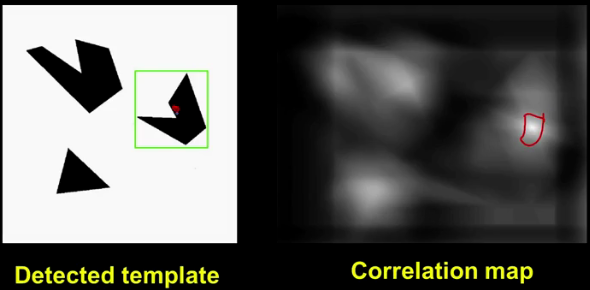这就是使用相关性进行检测的方法。
因为我们现在考虑的过滤器与其说是做平均或模糊化，还不如说是我们正在寻找的模板。
模板匹配实战
我敢打赌你一定很想自己做这件事。
这是我要你定义的函数，find_template_2D。
>> pkg load image;
>>
>> function [yIndex xIndex] = find_templete_2D(template, img)
>>     % TODO:
>> endfunction

以一个模板和一个图像作为输入，并返回在图像中找到模板的x和y坐标。
>> tablet = imread('tablet.png');
>> imshow(tablet);
我用图像来验证一下。我将从这个平板上剪下一个字形，作为我们的模板。
>> glyph = tablet(75:165, 150:185);
>> imshow(glyph);
记住，您希望找到模板左上角在图像中的位置。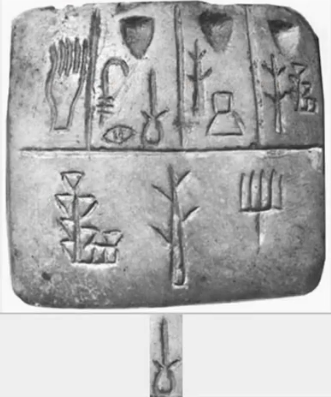还要注意返回坐标y x的顺序。
我们一起来做一下这个有趣的技术：
和之前一样，我们使用归一化互相关。
由于图像是一个二维矩阵，我们需要在寻找最大值时要仔细。
简单地写max (c)就可以计算列上的最大值。用单个冒号索引c可以使其变平。因此，max (c冒号)将计算图像宽最大值。
我们使用一个稍微不同的技巧来找到这个最大值发生的位置。
find函数直接返回行和列，即所需位置的y和x坐标。
记住，这些是相关系数矩阵的原始指数。
我们需要通过沿着适当的维度减去模板的大小并加上一个来计算偏移量。
>> pkg load image;
>>
>> function [yIndex xIndex] = find_templete_2D(template, img)
>>      c = normxcorr2(template, img);
[yRaw xRaw] = find(c == max(c(:)));
yIndex = yRaw - size(template, 1) + 1;
xIndex = xRaw - size(template, 2) + 1;
>> endfunction
>>
>> imshow(tablet);
>> glyph = tablet(75:165, 150:185);
>> imshow(glyph);
>>
>> [y x] = find_Template_2D(glyph, tablet);
>> disp([y x]);
运行代码，看看y和x的值。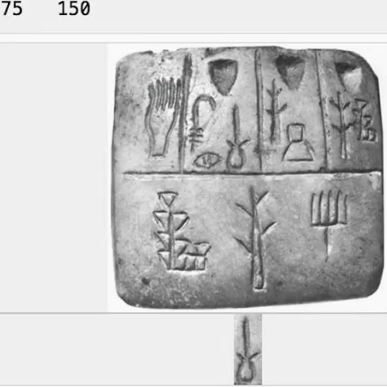75 150。
那不是我们裁剪象形文字的地方吗?
实际上，这些是左上角的坐标。
我们可以做一些额外的绘图使结果更直观。
在这里，我们首先显示原始图像，然后我们在它上面绘制一个红色加号，在那里找到了模板。
>> pkg load image;
>>
>> function [yIndex xIndex] = find_templete_2D(template, img)
>>      c = normxcorr2(template, img);
[yRaw xRaw] = find(c == max(c(:)));
yIndex = yRaw - size(template, 1) + 1;
xIndex = xRaw - size(template, 2) + 1;
>> endfunction
>>
>> imshow(tablet);
>> glyph = tablet(75:165, 150:185);
>> %imshow(glyph);
>>
>> [y x] = find_Template_2D(glyph, tablet);
>> disp([y x]);
>>
>> colormap('gray'), imagesc(tablet);
>> hold on;
>> plot(x, y, 'r+', 'markersize', 16);
>> hold off;
由于这不是一个完全交互式的环境，我们的绘图能力有限。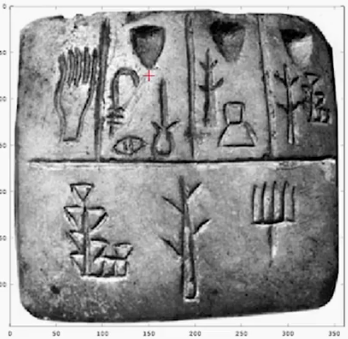在MATLAB或Octave的本地安装中，您可以使用更多的方法，比如在找到模板的地方绘制一个矩形。

——学会编写自己的代码，才能练出真功夫。
展开全文• 《基于Matlab 图像匹配――模板匹配》由会员分享，可在线阅读，更多相关《基于Matlab 图像匹配――模板匹配(8页珍藏版)》请在人人文库网上搜索。1、课程名称 数字图像处理 实验序号 实验8 实验名称 图像匹配模板匹配...

《基于Matlab 图像匹配――模板匹配》由会员分享，可在线阅读，更多相关《基于Matlab 图像匹配――模板匹配(8页珍藏版)》请在人人文库网上搜索。
1、课程名称 数字图像处理 实验序号 实验8 实验名称 图像匹配模板匹配 实验地点 综B207 实验学时 2 实验类型 验证性 2017年 10 月 16 日一、实验目的及要求在机器识别物体的过程，常需把不同传感器或同一传感器在不同时间，不同成像条件下对同一景物获取的两幅或多幅图像在空间上对准，或根据已知模式到另一幅图中寻找相应的模式，这就叫做匹配。模板匹配是一种最原始、最基本的模式识别方法。利用模板匹配可以在一幅图像中找到已知的物体。这里的模板指的是一幅待匹配的图像，相当于模式识别的模式。基本要求如下:(1).进行匹配的两幅图像为JPG格式或BMP格式。(2).能够进行对两幅数字图像的匹配。(3。
2、)采用交互式程序对图像进行匹配。二、实验原理与内容模板匹配是指用一个较小的图像，即模板与源图像进行比较，以确定在源图像中是否存在与该模板相同或相似的区域，若该区域存在，还可确定其位置并提取该区域。模板匹配常用的一种测度为模手术台与源图像对应区域的误差平方和。设f(x,y)为MN的源图像，t(j,k)为JK(JM,KN)的模板图像,则误差平方和测度定义为:DS(x,y)称为源图像中与模板对应区域的能量，它与像素位置(x,y)有关，但随像素位置(x,y)的变化，DS(x,y)变化缓慢。DST(x,y)模板与源图像对应区域的互相关，它随像素位置(x,y)的变化而变化，当模板t(j,k)和源图像中对应。
3、区域相匹配时取最大值。DT(x,y)称为模板的能量，它与图像像素位置(x,y)无关，只用一次计算便可。显然，计算误差平方和测度可以减少计算量。基于上述分析，若设DS(x,y)也为常数，则用DST(x,y)便可进行图像匹配，当DST(x,y)取最大值时，便可认为模板与图像是匹配的。但假设DS(x,y)为常数会产生误差，严重时将无法下确匹配，因此可用归一化互相关作为误差平方和测度，其定义为：模板匹配的示意图如图图2.1所示，其中假设源图像f(x,y)和模板图像t(k,l)的原点都在左上角。对任何一个f(x,y)中的(x,y),根据上式都可以算得一个R(x,y).当x和y变化时，t(j,k)在源图像。
5、eitu);%将模板图灰度化pipei_height,pipei_width=size(pipeitu2gray);yuantu_height,yuantu_width=size(yuantu2gray);imshow(yuantu);%显示原图像hold on;for i=1:yuantu_height-pipei_heightfor j=1:yuantu_width-pipei_width temp_picture=imcrop(yuantu2gray,j,i,pipei_width-1,pipei_height-1);r=corr2(temp_picture,pipeitu2gray);。
6、%取得相关系数if r0.95 %规定值为0.95%下面用plot函数在原图的坐标系上画出匹配区域plot(j:j+pipei_width,i,b);plot(j:j+pipei_width,i+pipei_height,b);plot(j,i:i+pipei_height,b);plot(j+pipei_width,i:i+pipei_height,b);endendend这是在原图上截取一部分作为模板，经过比较长的运算，终于可以在原图像上匹配到的区域五、测试/调试及实验结果分析通过代码，可以看到在原图像找到了匹配区域，并且可以画上了对应的框。六、实验结论与体会结论：通过本次实验学会了如何在原图像上截取部分作为匹配模板。由于本次读入的图像大概为250k，相对有点大，所以经过了比较长的时间才将匹配区域找到。因此我觉得，提高效率的有连个，一是先把图像经过压缩再进行匹配，二是优化算法，提高代码的执行率。

展开全文• 二值化图像处理 将得到车牌区域进行二值化，然后利用bwmorph函数对二值图像应用形态学操作，经过擦除，再次裁剪，得到更好只具有黑白色彩的车牌图像 字符分割 将计算得到车牌区域的彩色分割后的图象，对白色进行...人工智能 机器学习 字符识别
• 好文网为大家准备了关于MATLAB函数模板范文,好文网里面收集了五十多篇关于好MATLAB函数模板好文,希望可以帮助大家。更多关于MATLAB函数模板内容请关注好文网篇一：MATLAB图象处理函数MATLAB图象处理函数1.图象1如何...
• 引言：在利用显微镜观测量物体的长度时，其精度非常高，然而，其行程却有限。一个测量较长物体的长度时，... 模板匹配：对于上面的图像，可以明显看出其往右移了，但对于精密测量来讲，求其移动的距离并精确到像素级非
• 在一个图像区域中，物体检测的一种最基本的方法是通过模板匹配来进行。...本次试验的模板匹配的思想：利用快速傅里叶变化的一个重要特性，即连个函数的卷积的傅立叶变换等于两个函数的傅立叶变换的乘积。
• 较为简单的模板匹配函数，应用matlab写的，已经测试过了，很简单方便，适合初级学习，接口注释全部有，喜欢的可以自己下载。
• NCC多尺寸模板匹配MATLAB模板匹配NCC运行结果具体代码 模板匹配 模板匹配首先给出一份模板，通过对于目标图像中的检索，找到目标图像中区域与模板最为契合的部分。 再本次程序中，流程如下： 获得模板与原图像的 ...计算机视觉
• ## 基于matlab+模板匹配的车牌识别

万次阅读 多人点赞 2018-07-30 13:29:41
这里我把图像的长度分成15等分，出去中间的圆点只占一份之外，其余部分的字符都是占用两个位置，这样不仅能去除圆点，还可以顺利的分割出字符，分割完之后的字符进行48*24的归一化处理，方便之后的模板匹配。...车牌识别 程序 源代码
• 阈值分割后基于形状做的模板匹配，使用最大熵阈值分割法，效果优于otsu。
• MATLAB实现模板匹配算法（手写数字和车牌数据） MOOC地址：https://www.icourse163.org/learn/BIT-1206703821?tid=1457771445#/learn/announce 课程数据集：链接：https://pan.baidu.com/s/1YiWy6cQvmbCiaSU1-wtrLw ...模式识别
• 汉字模板为200+，详见ziku.mat 主文件：main.m 有用到matlab2014a的函数，注意
• 仅作为学习和练习的用途，不作为毕设以及其他商业用途。
• 1 字符识别简介 字符识别是车牌识别中很重要的一部分，在模式识别中也扮演的很重要的角色。当然，我们可以用很多方法...当然基于模板匹配也是一种方法。模板匹配既简单有具有实效性。其中关键在于模板的选取。好的模板
• 给定一个模板作为二值边缘图像，该函数在图像中找到与模板匹配的对象。 即使模板和对象处于不同的方向，这项工作也能正常工作。 匹配是通过旋转模板并将其以各种角度与图像匹配来完成的，返回最佳匹配。 模板与图像...
• 相关系数计算不是使用内置函数 ... 函数 corr 用于模板匹配目的相对较慢，并且还需要额外考虑控制边界和选择帧图像上的感兴趣区域。 但是，通过使用 conv2，模板匹配速度得到了加快，运行时间也减少到了一个合理的值。
• 带旋转的简单模板匹配在图像中找到适合模板的对象。图像中对象的方向不必与模板相同。 模板以各种旋转与图像匹配，并选择最佳匹配。 该函数使用模板和图像的canny边缘图像之间的互相关来确定最佳匹配。 MAIN_find_...图像滤波
•图像配准
• 【车牌识别】基于模板匹配算法实现车牌识别matlab源码 1 模板匹配算法介绍 随着社会经济的发展,车辆的数量在急剧的增加,交通问题日益突出,这使得智能交通(Intelligent Transportation System,简称ITS)系统成为研究...算法 自动驾驶
• 如果要在一幅图像中寻找已知物体，最常用且最简单的方法之一就是...% 基于最小距离分类器的模板匹配 % 寻找图片中与已知模板的匹配区域 % date:2016-4-15 % author:fengyun %%%%%%%%%%%%%%%%%%%%%%%%%%%%%%%%%%%%%%%%图像处理 opencv
• 通过计算机中 的 Matlab 软件对图片进行处理,包括二值图像的转换、数学形态学的膨胀、边 缘检测等,并提取图像的特征值通过模板匹配方法在后台模板库进行搜索完成最 ......考虑到每种方法的特点以及待跟踪目标具有...
• 1.模板匹配基本原理概述 当我们比较两幅图像的时候，首先面对的基本问题是：什么时候两幅图像才是一样或比较相似的，这两幅图像的相似程度如何衡量？当然，比较一般的方法是，当两幅图像的所有像素灰度值一样的...自动驾驶 人工智能
• 在模式识别的各种方法中，模板匹配是最容易的一种，其数学模型易于建立，通过模板匹配对数字图像模式识别有助于我们了解数学模型在数字图像中的应用。 2 模板匹配算法 2.1 相似性测度求匹配 模板匹配的实际操作思路...算法
•  模板匹配是在图像中寻找目标的方法之一。Come On, Boy.我们一起来看看模板匹配到底是怎么回事。   模板匹配的工作方式  模板匹配的工作方式跟直方图的反向投影基本一样，大致过程是这样的：通过在输入图像上滑动......

# matlab模板匹配函数matlab 订阅